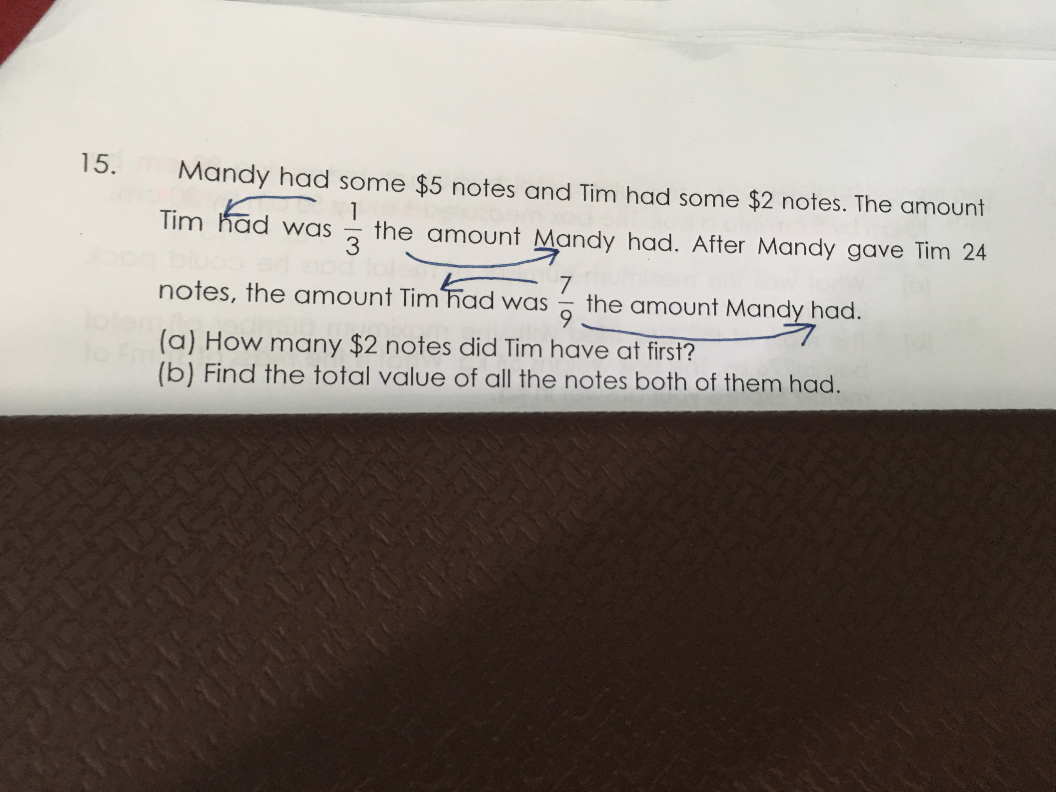# QuestionHi, P6 math question. Anyone can help

(i)

before

T: M = 1:3 (total 4 )

after

T:M = 7:9(total 16)

Mandy gave 24 \$5 notes to tim.

( total value unchanged)

thus we will rewrite the before ratio as T: M = 4:12

thus 3u = 24×5=120

1u = 40

4u =160

thus tim has 160/2 = 80 \$2 notes at first.

(ii)total value = 16×40=\$640

0 Replies 1 Like

(a)
Before —
Tim : Mandy : Total
1u : 3u : 4u
4u : 12u : 16u

After —
Tim : Mandy : Total
7u : 9u : 16u

24 x 5 = 120
12u – 9u = 3u ——- 120
1u ——- 120/3 = 40

4u ——- 4 x 40 = 160
160/2 = 80

(b)
16u ——- 16 x 40 = 640

Ans (a) 80 \$2-notes; (b) \$640.

0 Replies 1 Like